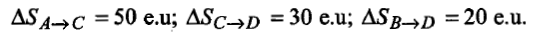If S$°$ for H2, Cl2 and HCl are 0.13, 0.22 and 0.19 kJ K-1mol-1 respectively. The total change in standard entropy for the reaction, H2 + Cl2 $\to$2HCl is:

(a) 30 J K-1mol-1                         (b) 40 J K-1mol-1

(c) 60 J K-1mol-1                         (d) 20 J K-1mol-1

Concept Questions :-

Spontaneity and Entropy
High Yielding Test Series + Question Bank - NEET 2020

Difficulty Level:

The direct conversion of A to B is difficult and thus it is converted by path A$\to$C$\to$D$\to$B. GivenWhere e.u. is the entropy unit, then $∆{\mathrm{S}}_{\mathrm{A}\to \mathrm{B}}$ is:

(a) +60 e.u                                       (b) +100 e.u

(c) -60 e.u                                        (d) -100 e.u

Concept Questions :-

Hess's Law
High Yielding Test Series + Question Bank - NEET 2020

Difficulty Level:

In a flask, colourless N2O4(g) is in equilibrium with brown coloured NO2(g). At equilibrium when the flask is heated to 100$°$C, the brown colour deepens and on cooling it becomes coloured. Which statement is incorrect about this observation?

(a) The $∆$H for the reaction N2O4(g)$⇌$ 2NO2(g) is +ve

(b) Paramagnetism increases on heating

(c) The $∆$H-$∆$U at 100$°$C is equal to 200 cal

(d) Dimerisation is reduced on heating

Concept Questions :-

First Law of Thermodynamics
High Yielding Test Series + Question Bank - NEET 2020

Difficulty Level:

Enthalpy of the reaction,

CH4(g) + 1/2 O2(g) $\to$CH3OH (l), is negative. If enthalpy of combustion of CH4 and CH3OH are x and y respectively, then which relation is correct?

(a) x>y         (b) x<y          (c) x=y           (d) x$⩾$y

High Yielding Test Series + Question Bank - NEET 2020

Difficulty Level:

In the reaction, $∆$H and $∆$S both are positive. In which of the following cases, the reaction would not be spontaneous?

(a) $∆$H>T$∆$S                              (b) $∆$S=$∆$H/T

(c) $∆$H=T$∆$S                               (d) All of these

High Yielding Test Series + Question Bank - NEET 2020

Difficulty Level:

When an ideal gas is compressed adiabatically and reversibly, the final temperature is:

(a) higher than the initial temperature

(b) lower than the initial temperature

(c) the same as the initial temperature

(d) dependent on the rate of compression

Concept Questions :-

First Law of Thermodynamics
High Yielding Test Series + Question Bank - NEET 2020

Difficulty Level:

1 litre-atmosphere is equal to:

(a) 101.3 J                           (b) 24.206 cal

(c) 101.3 x 107 erg               (d) all of these

Concept Questions :-

First Law of Thermodynamics
High Yielding Test Series + Question Bank - NEET 2020

Difficulty Level:

The standard change is Gibbs energy for the reaction,

H2O$⇄$H+ + OH- at 25$°$C is:

1. 100 kJ                         2. -90 kJ

3. 90 kJ                           4. -100 kJ

Concept Questions :-

Spontaneity and Entropy
High Yielding Test Series + Question Bank - NEET 2020

Difficulty Level:

The entropy change in the fusion of one mole of a solid melting at 27$°$C (latent heat of fusion is 2930 J mol-1) is

(a) 9.77 JK-1mol-1                (b) 10.73 JK-1mol-1

(c) 2930 JK-1mol-1                (d) 108.5 JK-1mol-1

Concept Questions :-

Spontaneity and Entropy
High Yielding Test Series + Question Bank - NEET 2020

Difficulty Level:

The maximum work done in expanding 16 g oxygen at 300 K and occupying a volume of 5 dm3 isothermally until the volume becomes 25 dm3 is:

(a) 2.01 x 103 J                       (b) +2.81 x 103 J

(c) 2.01 x 10-3 J                      (d) +2.01 x 10-6 J

Concept Questions :-

Internal Energy and work done